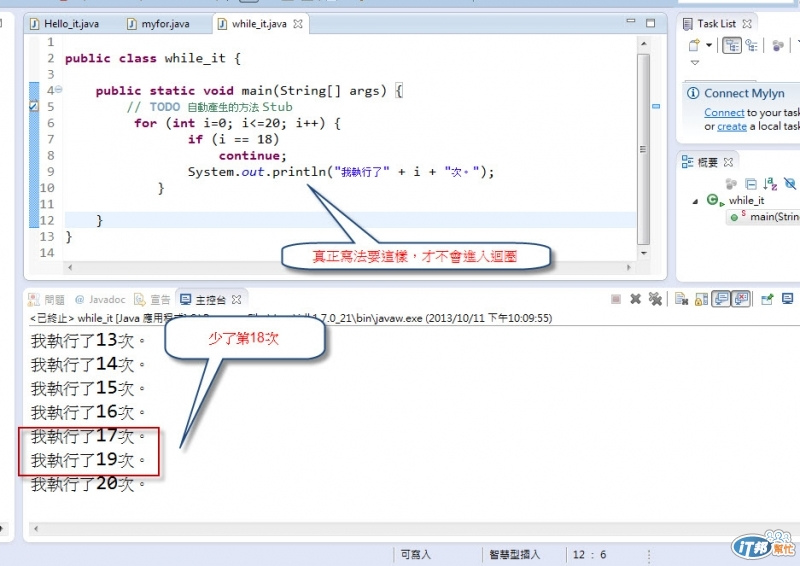DAY 26
3

程式設計師之入門基礎必備知識(26)-ECLIPSE JAVA(下)

JAVA程式語言暫時完結拉，雖然有點過於倉促，但是只要學習到這邊的精華，就夠妳在JAVA上能夠闖上好幾年，因為變化一定是無止境的，但是都回歸於基礎來的，以上是我自導自編，針對菜鳥入門程式設計師需要學習心態與哪些基礎技巧。

while迴圈public class while_it {

public static void main(String[] args) {
// TODO 自動產生的方法 Stub
int i= 0 ,x,z;
while(i <= 20){
System.out.println("我執行了"+i+"次");
i++;
}
}

}public class while_it {

public static void main(String[] args) {
// TODO 自動產生的方法 Stub
int i= 0 ,x,z;
while(i <= 20){
System.out.println("我執行了"+i+"次");
if(i == 3)break;
i++;
}
}

}public class while_it {

public static void main(String[] args) {
// TODO 自動產生的方法 Stub
int i= 0 ,x,z;
while(i <= 20){
System.out.println("我執行了"+i+"次");
if(i == 3)continue;
i++;
}
}
}public class while_it {

public static void main(String[] args) {
// TODO 自動產生的方法 Stub
for (int i=0; i<=20; i++) {
if (i == 18)
continue;
System.out.println("我執行了" + i + "次。");
}

}
}